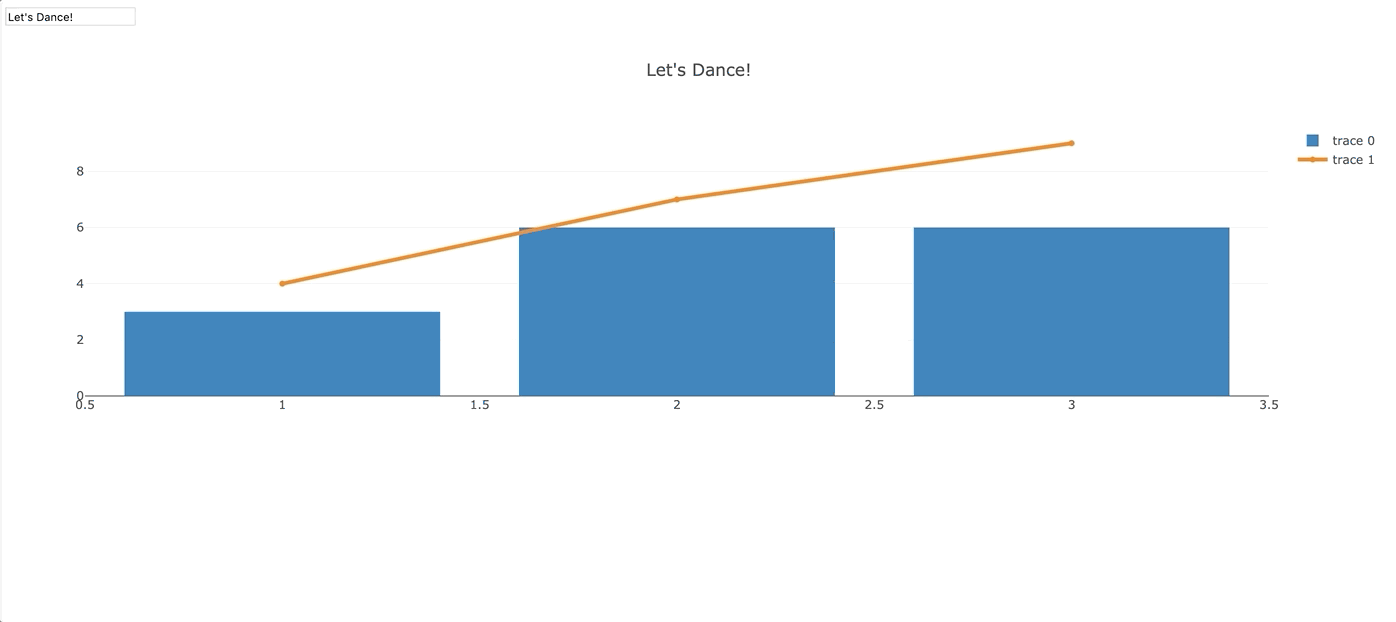# Dash for R

## Installation

https://dashr.plotly.com/installation

🛑 Make sure you're on at least version `3.0.2` of R. You can see what version of R you have by entering `version` in the R CLI. CRAN is the easiest place to download the latest R version.

As of 2020-06-04, dash and the currently released versions of all core component libraries are available for download via CRAN! Installing `dash` and its dependencies is as simple as

```install.packages("dash")
```

Users who wish to install (stable) development versions of the package as well as Dash components from GitHub may instead use `install_github` and specify the development branch:

```install.packages(c("fiery", "routr", "reqres", "htmltools", "base64enc", "plotly", "mime", "crayon", "devtools"))

# installs dashHtmlComponents, dashCoreComponents, and dashTable
# and will update the component libraries when a new package is released
```

Then, to load the packages in R:

```library(dash)
library(dashHtmlComponents)
library(dashCoreComponents)
library(dashTable)
```

That's it!

## Getting Started

https://dashr.plotly.com/getting-started

The R package dash makes it easy to create reactive web applications powered by R. It provides an R6 class, named `Dash`, which may be initialized via the `new()` method.

```library(dash)
library(dashHtmlComponents)
library(dashCoreComponents)

app <- Dash\$new()
```

Similar to Dash for Python and Dash for Julia, every Dash for R application needs a layout (i.e., user interface) and a collection of callback functions which define the updating logic to perform when input value(s) change. Take, for instance, this basic example of formatting a string:

```app\$layout(
htmlDiv(
list(
dccInput(id = "inputID", value = "initial value", type = "text"),
htmlDiv(id = "outputID")
)
)
)

app\$callback(output = list(id="outputID", property="children"),
params = list(input(id="inputID", property="value"),
state(id="inputID", property="type")),
function(x, y) {
sprintf("You've entered: '%s' into a '%s' input control", x, y)
}
)

app\$run_server(showcase = TRUE)
```

Here the `showcase = TRUE` argument opens a browser window and automatically loads the Dash app for you.

## Hello world example using `dccGraph`

```app <- Dash\$new()

app\$layout(
htmlDiv(
list(
dccInput(id = "graphTitle",
value = "Let's Dance!",
type = "text"),
htmlDiv(id = "outputID"),
dccGraph(id = "giraffe",
figure = list(
data = list(x = c(1,2,3), y = c(3,2,8), type = "bar"),
layout = list(title = "Let's Dance!")
)
)
)
)
)

app\$callback(output = list(id = "giraffe", property = "figure"),
params = list(input("graphTitle", "value")),
function(newTitle) {

rand1 <- sample(1:10, 1)

rand2 <- sample(1:10, 1)
rand3 <- sample(1:10, 1)
rand4 <- sample(1:10, 1)

x <- c(1,2,3)
y <- c(3,6,rand1)
y2 <- c(rand2,rand3,rand4)

df = data.frame(x, y, y2)

list(
data =
list(
list(
x = df\$x,
y = df\$y,
type = "bar"
),
list(
x = df\$x,
y = df\$y2,
type = "scatter",
mode = "lines+markers",
line = list(width = 4)
)
),
layout = list(title = newTitle)
)
}
)

app\$callback(output = list(id = "outputID", property = "children"),
params = list(input("graphTitle", "value"),
state("graphTitle", "type")),
function(x, y) {
sprintf("You've entered: '%s' into a '%s' input control", x, y)
}
)

app\$run_server(showcase = TRUE)
```

## Screenshot of "Hello World" appGet A Weekly Email With Trending Projects For These Topics
No Spam. Unsubscribe easily at any time.
javascript (70,969
python (55,543
react (5,572
r (2,411
data-science (924
data-visualization (439
web-application (117
dash (100
plotly (69
plotly-dash (31